# Shapes

Have you noticed the shape of a pizza? It is round in shape.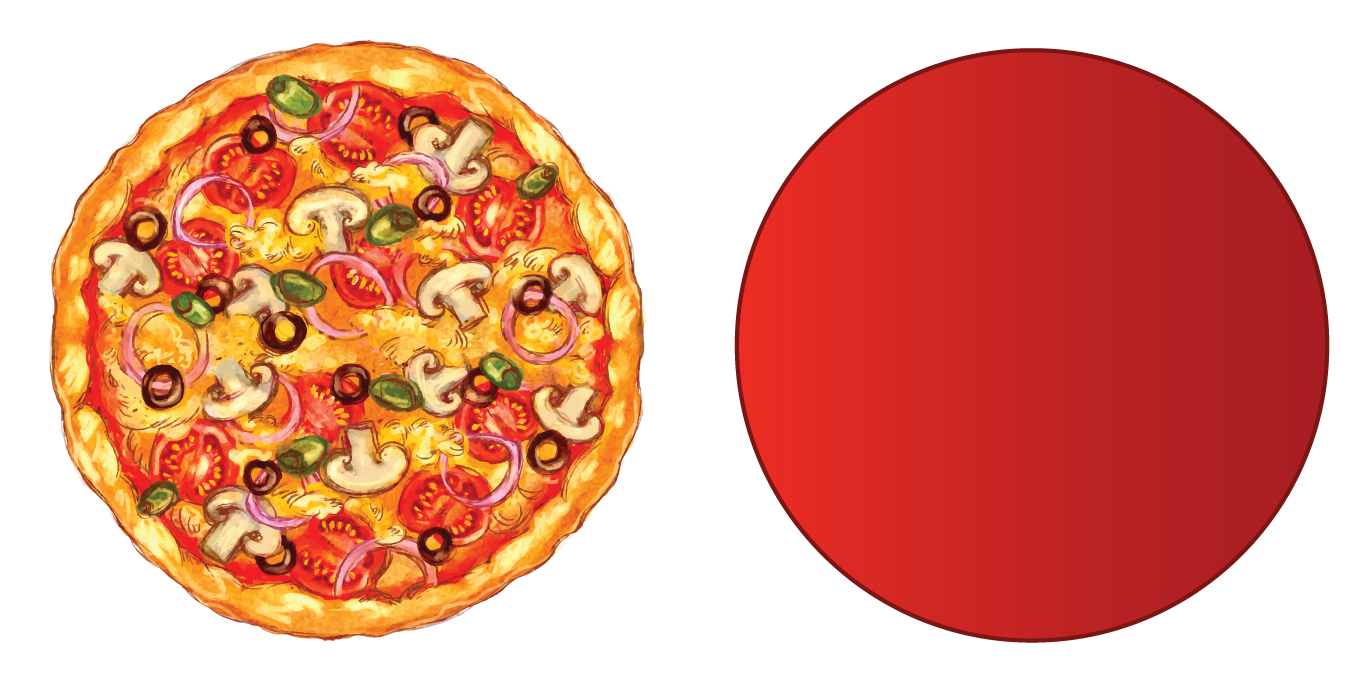If we cut out a slice from the pizza, the slice gets a triangular shape.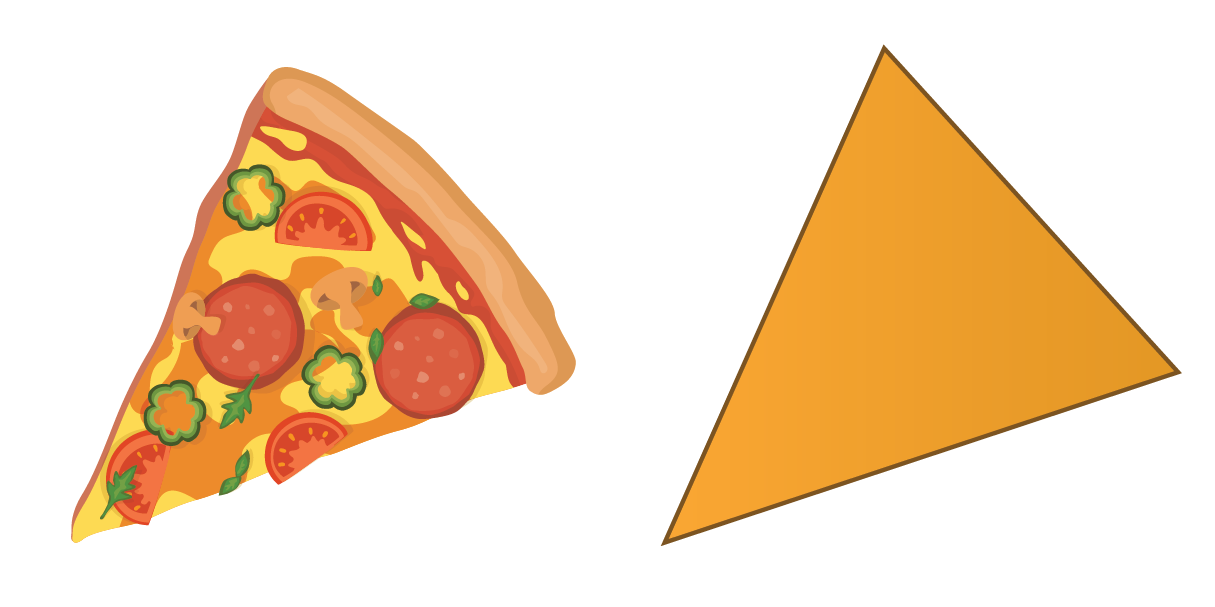In this lesson, you will learn all about shapes, types of shapes, and geometric shapes. Check out the interactive simulations to know more about the lesson and try your hand at solving a few interesting practice questions at the end of the page. So, what are you waiting for?

Let's begin!

## What are Shapes?

A shape can be defined as the boundary or outline of an object. It is the surface we see and does not depend on the size or the color of the object.

Everything around us has a different shape, such as the square, rectangle, or three-dimensional sphere. Have you seen the model of the Earth called the globe? What is the shape of this globe?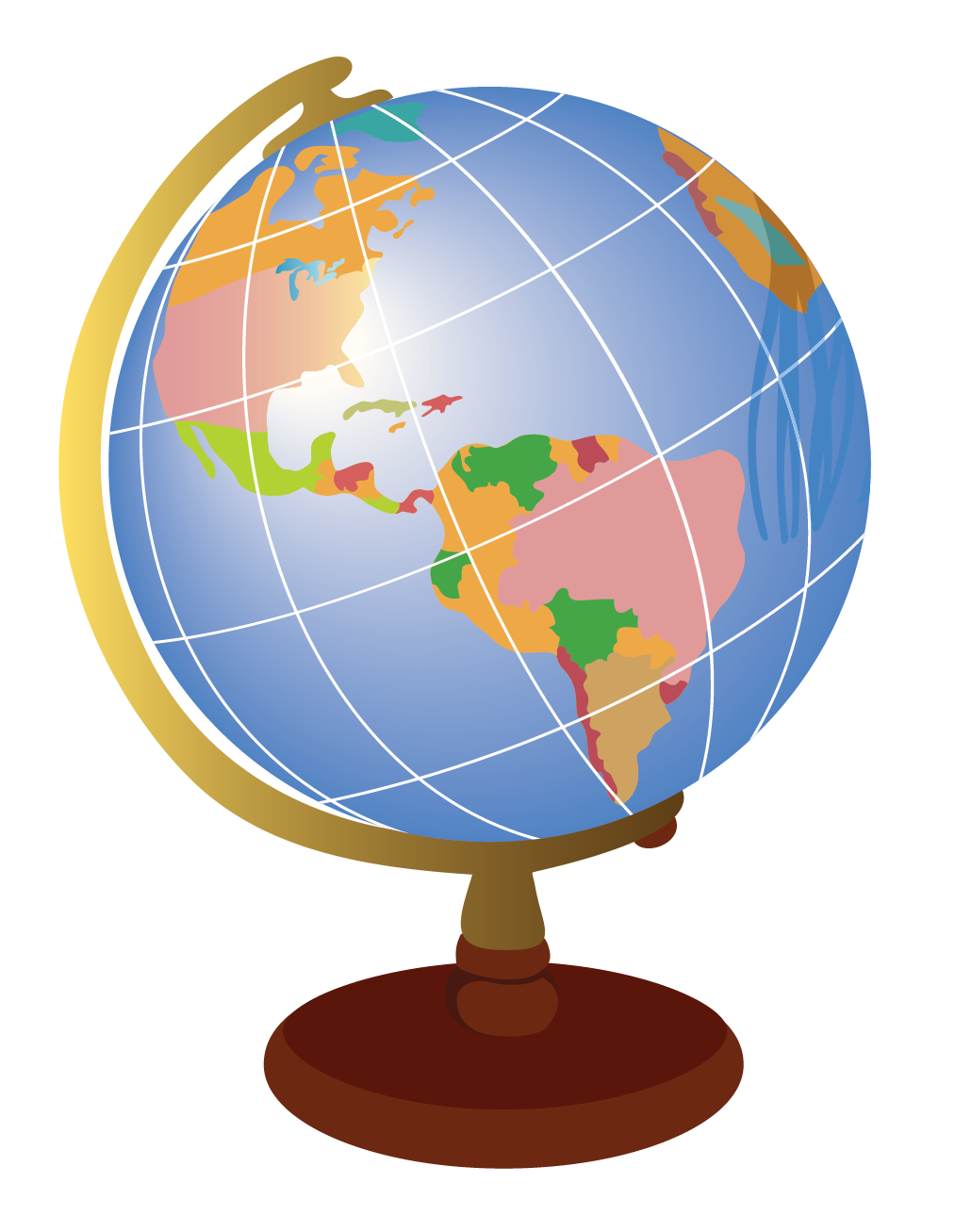It looks like a sphere.

Now, look at this pencil box.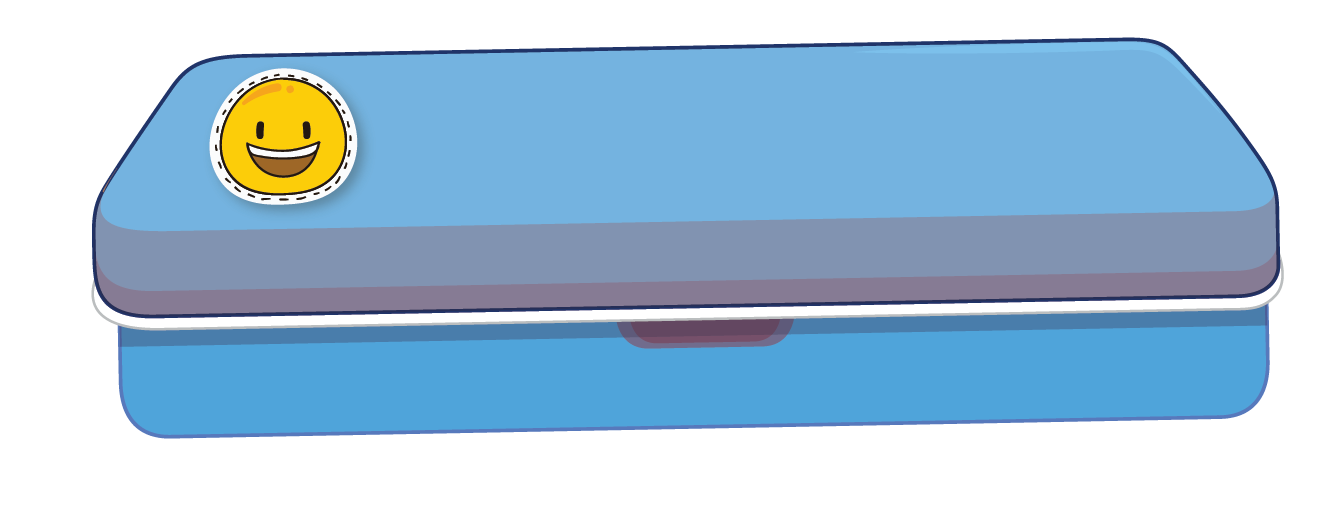It looks like a cuboid.

### Types of Shapes

Shapes define the boundary of an object and can be differentiated in many ways based on their properties.

Shapes can be of various types:

Closed Shapes

Closed shapes can be traced without any break. They start and end in the same place.

Let's look at these examples: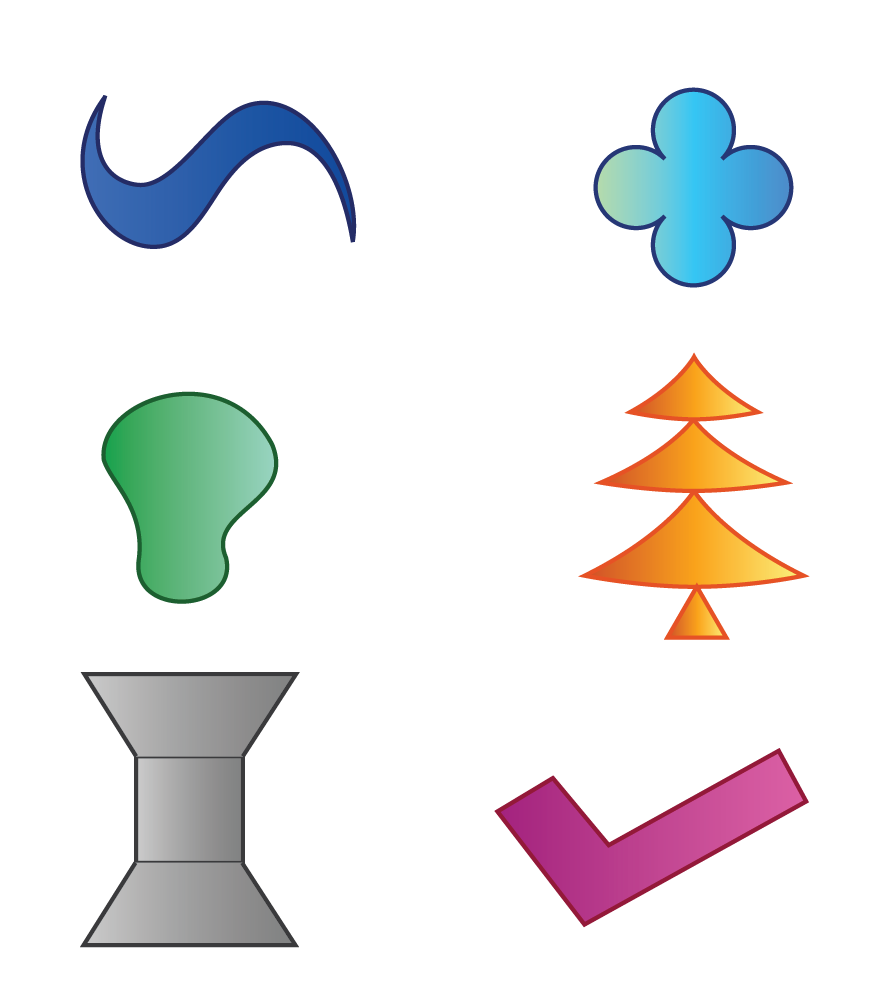Open Shapes

Open shapes are not continuous and are made up of line segments or curves which do not meet.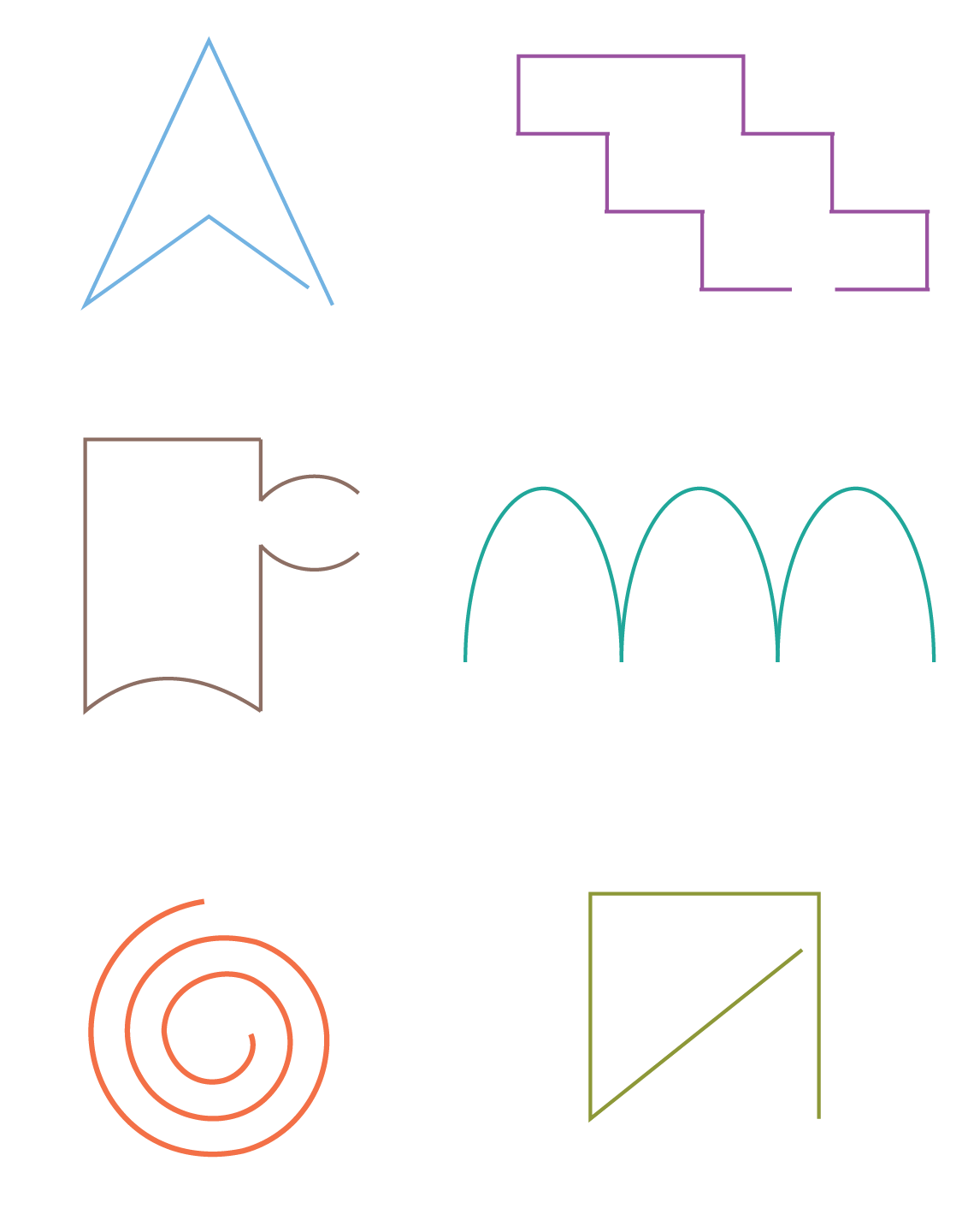Two-dimensional $$(2\text{D})$$ or three-dimensional $$(3\text{D})$$ shapes

A shape can be two-dimensional ($$2\text{D}$$) or three-dimensional ($$3\text{D}$$).

A square is a $$2\text{D}$$ shape, whereas, a cube is a $$3\text{D}$$ shape.

$$3\text{D}$$ shapes have a length, a width, and a height. You can learn more about it here.

$$2\text{D}$$ shapes, as the name suggests, have only two of these measurements.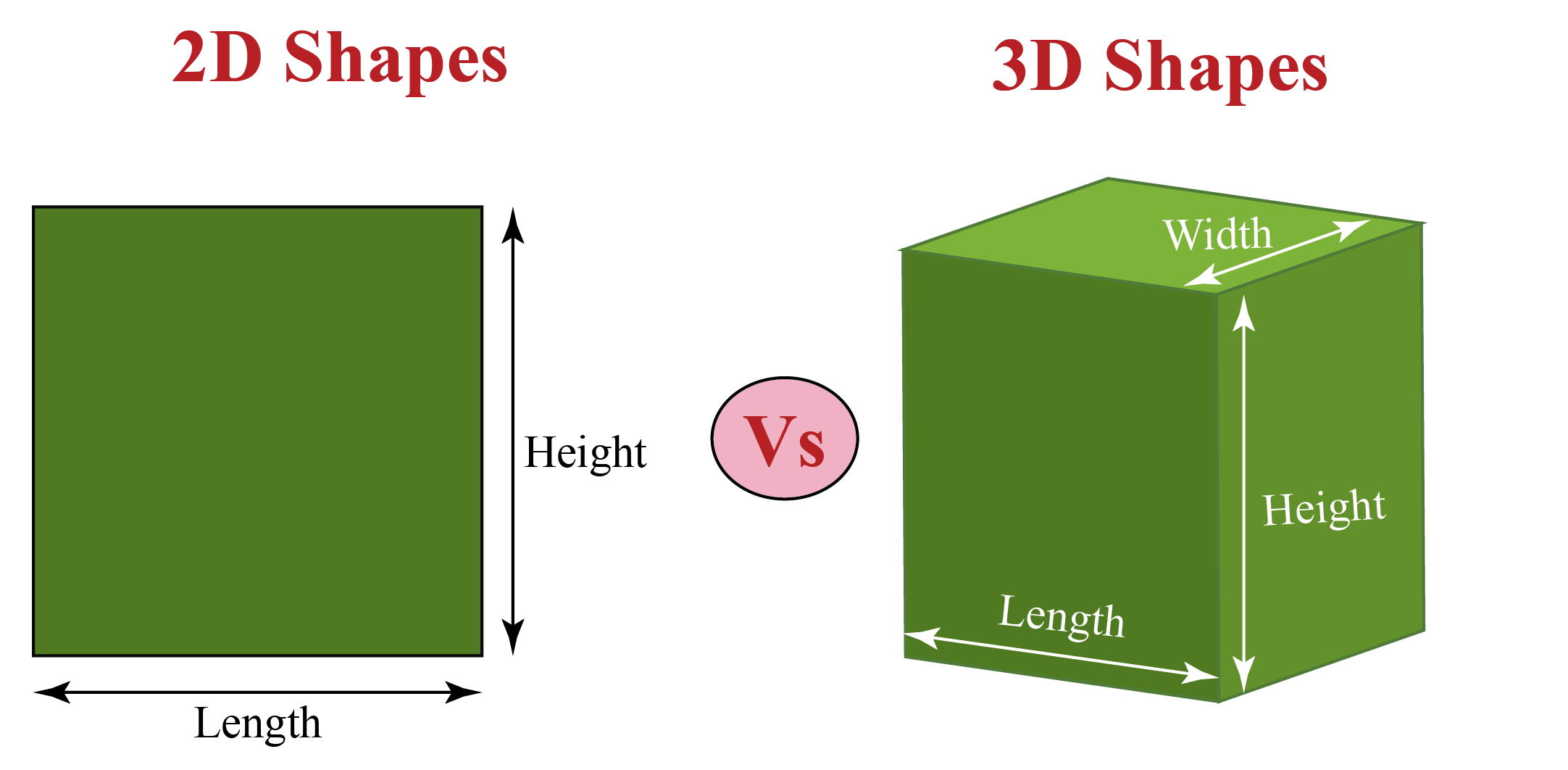The common $$2\text{D}$$ and $$3\text{D}$$ shapes are listed here in Table - 1 and Table - 2.

## List of Geometric Shapes

We know that shapes are made of straight lines or curved lines and they can be open or closed.

Lines are defined as a collection of points. In other words, many points put together to form a line.

They may form a straight line or a curved line.

Geometric shapes are closed shapes which are created by joining lines together.

Look at this shape below; this is made of four straight lines.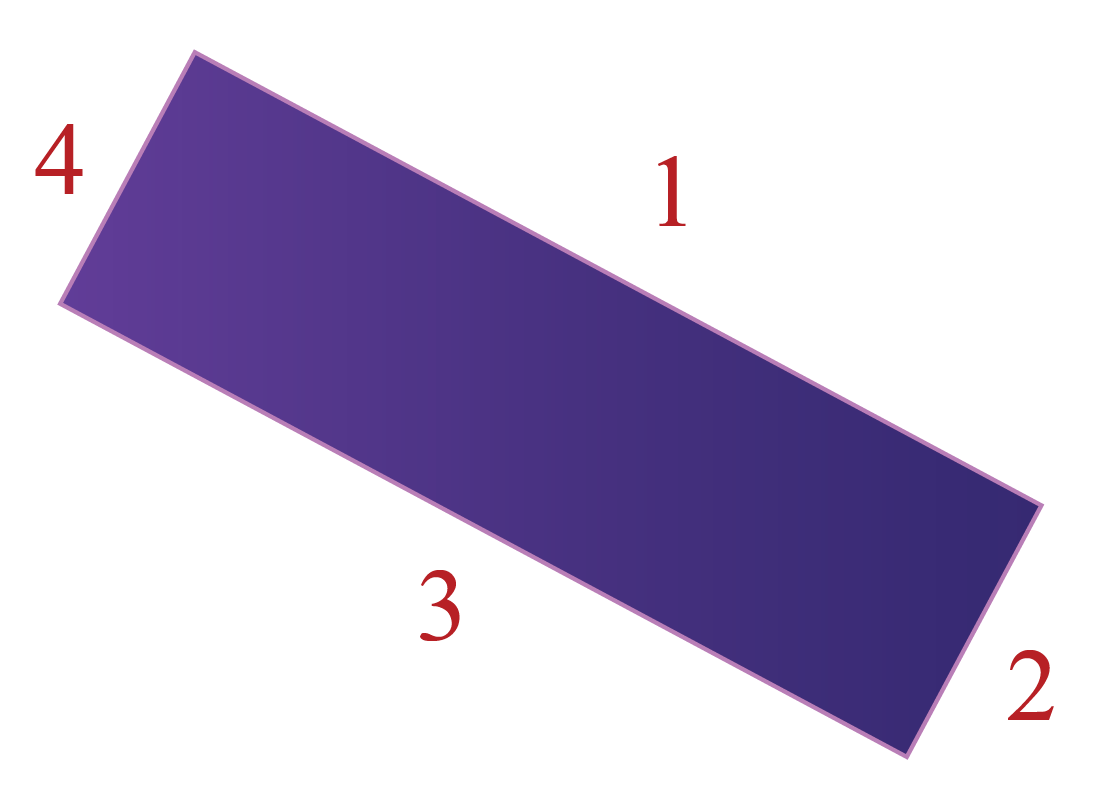Can you think of another quadrilateral shape?

Next, look at this shape: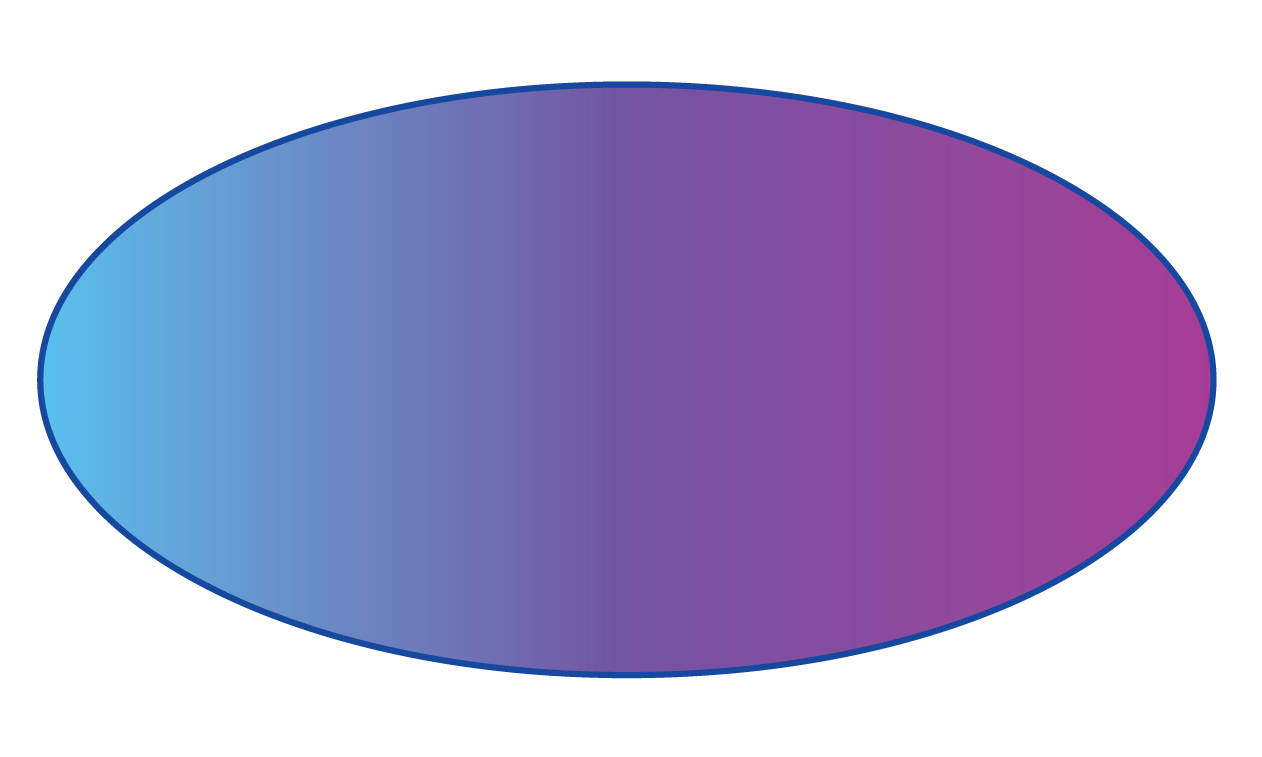It has no straight line, corner, or side to it. It is a closed shape made of curved lines.

This shape is called an ellipse.

## What are Two-Dimensional Shapes?

Observe this sheet of paper.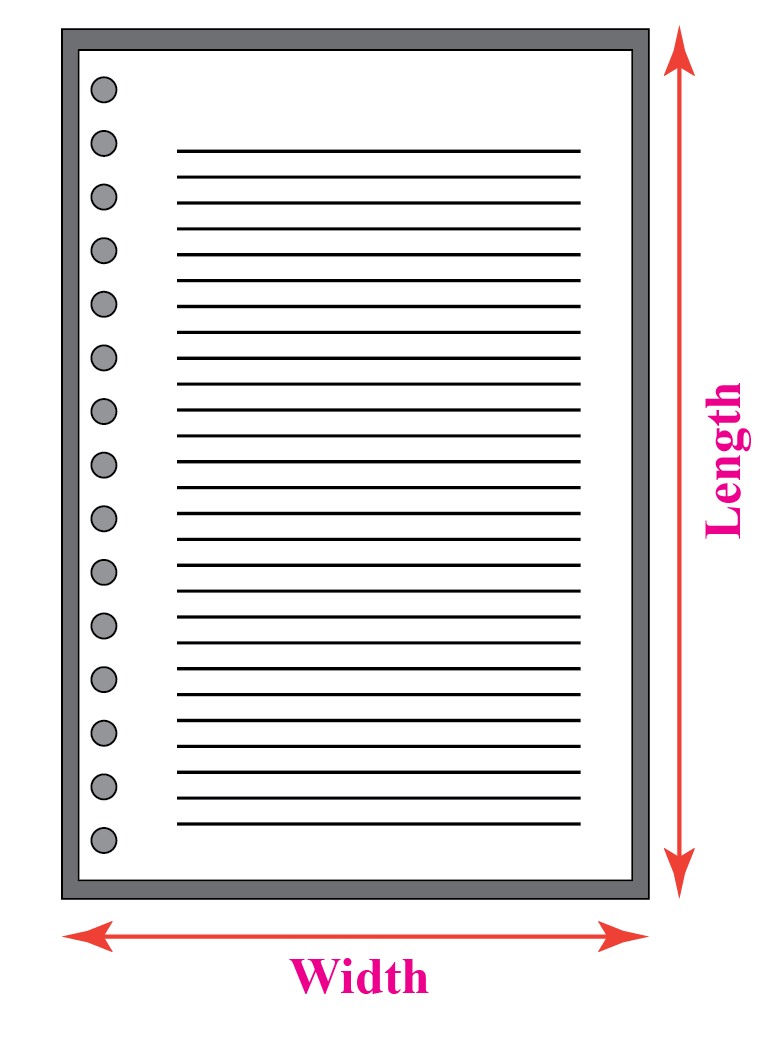It consists of a length and a width.

A two-dimensional shape does not have any depth.

The common $$2\text{D}$$ shapes we see are listed below:

Table - 1

 2D Shapes Name Number of sides Image Triangle 3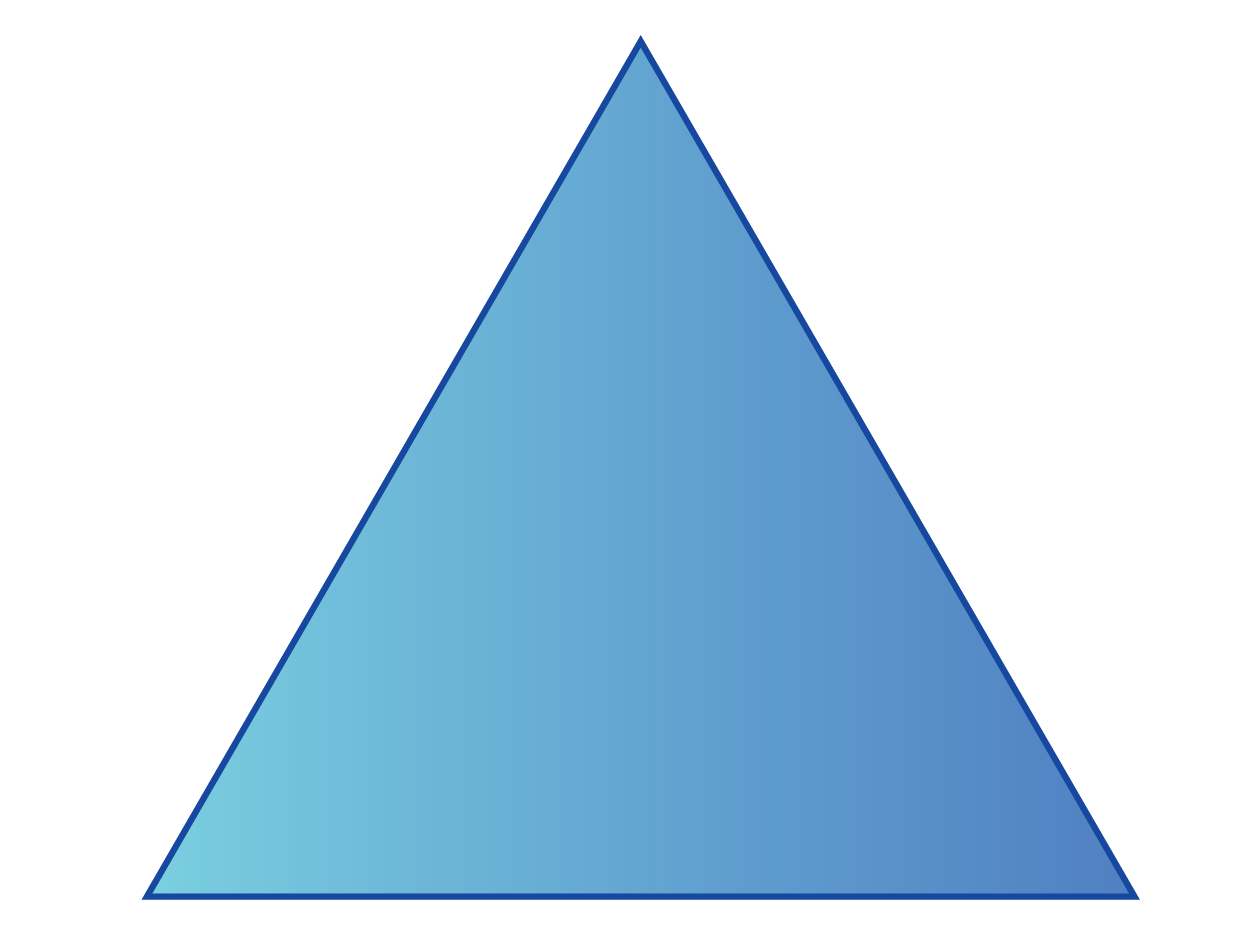Square 4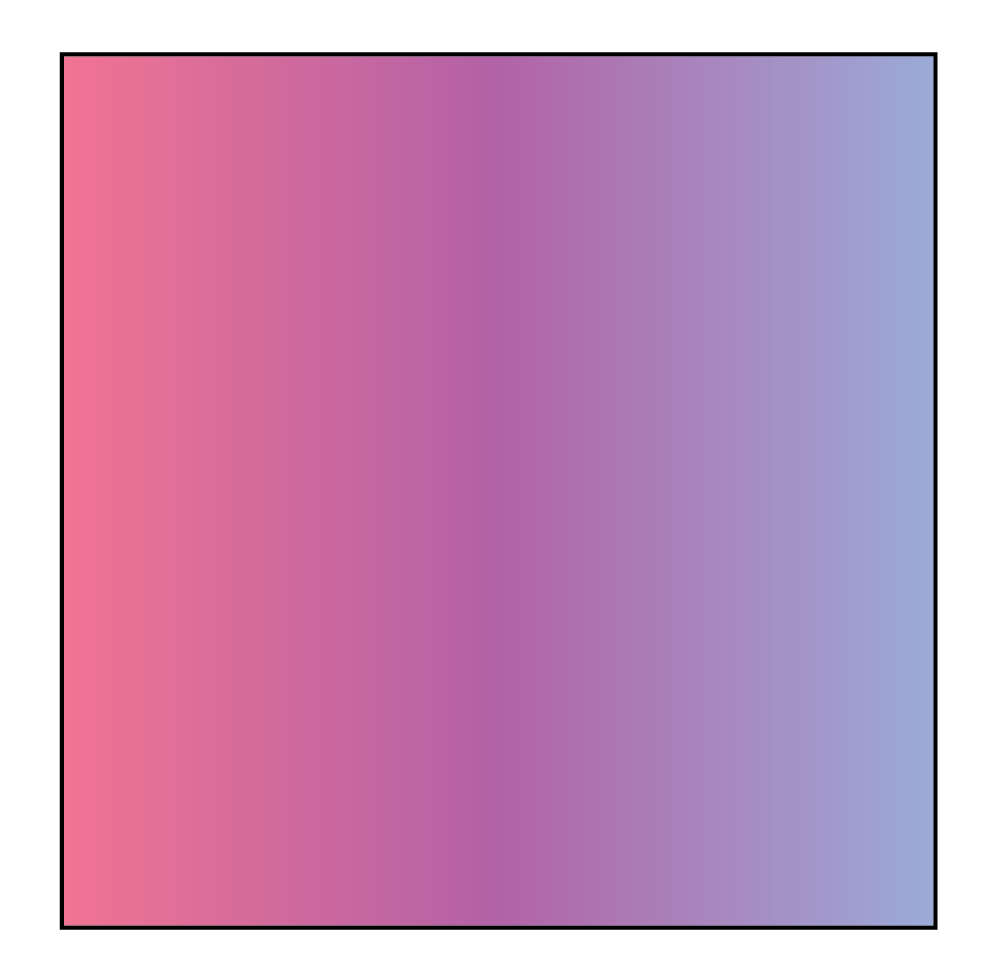Rhombus 4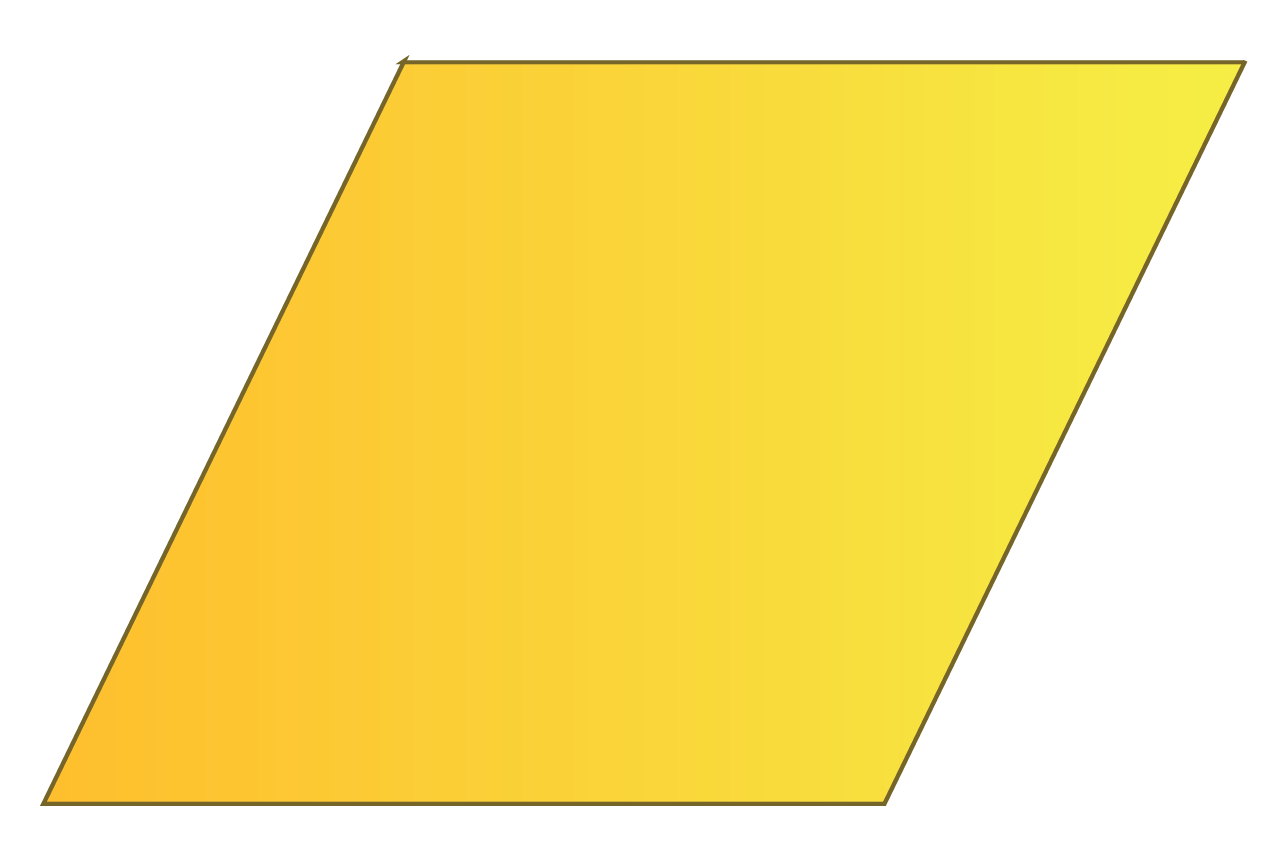Kite 4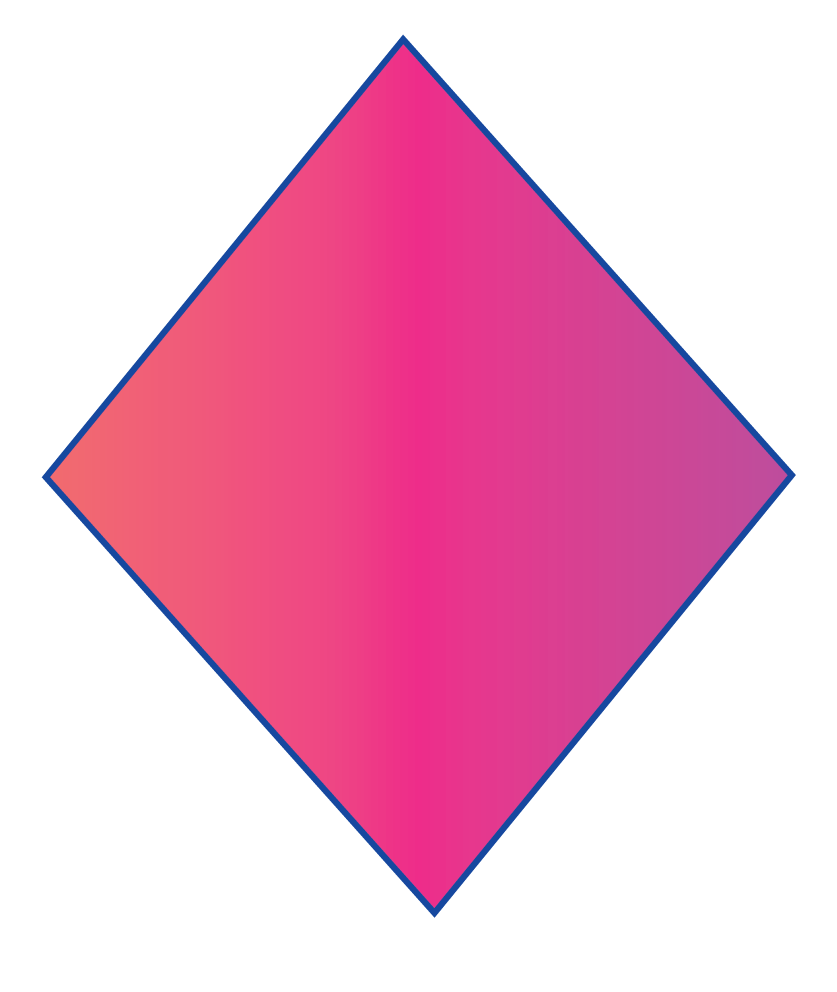Rectangle 4Trapezium 4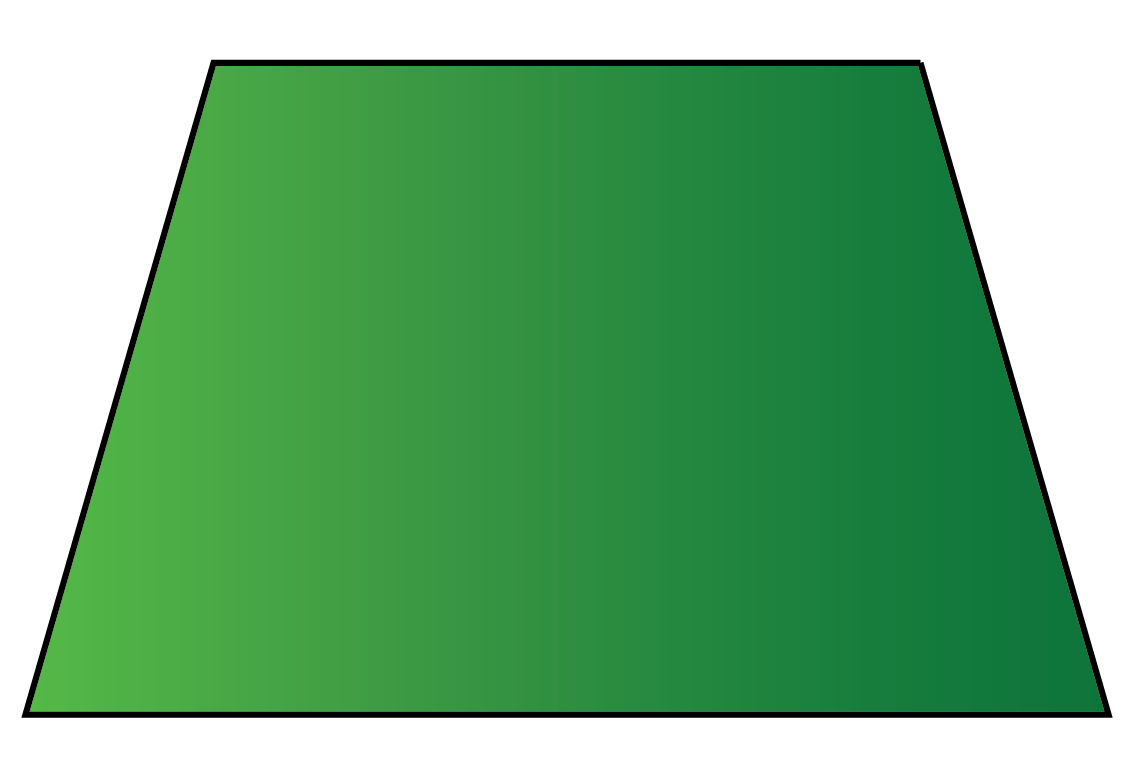Pentagon 5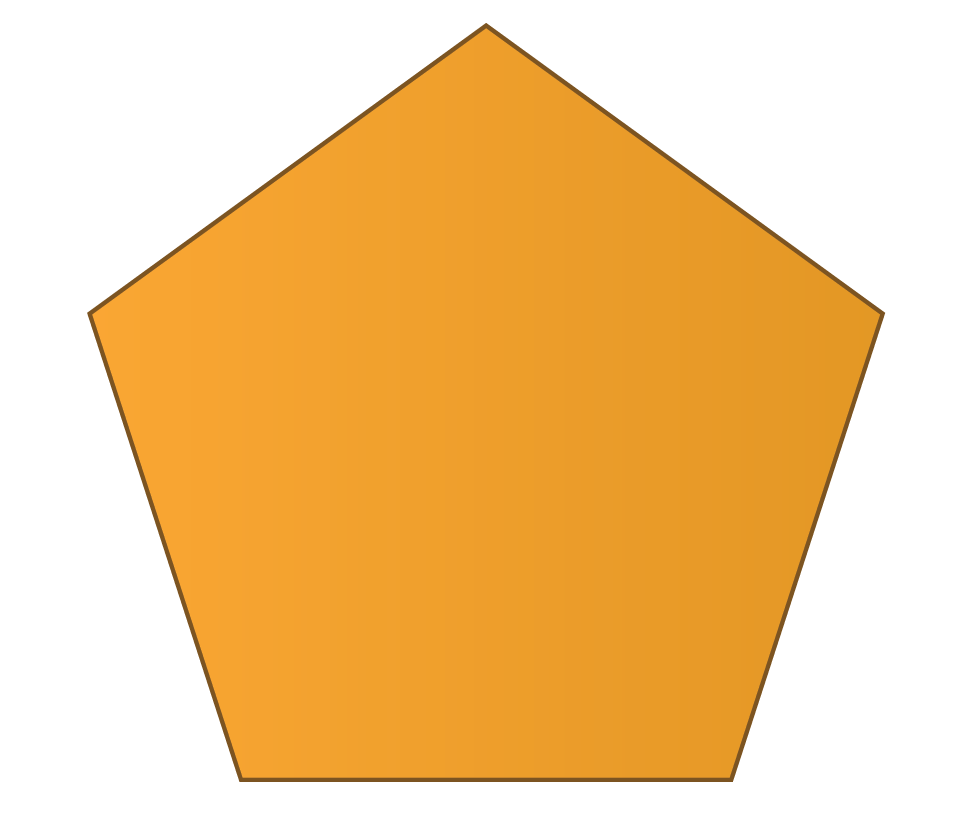Hexagon 6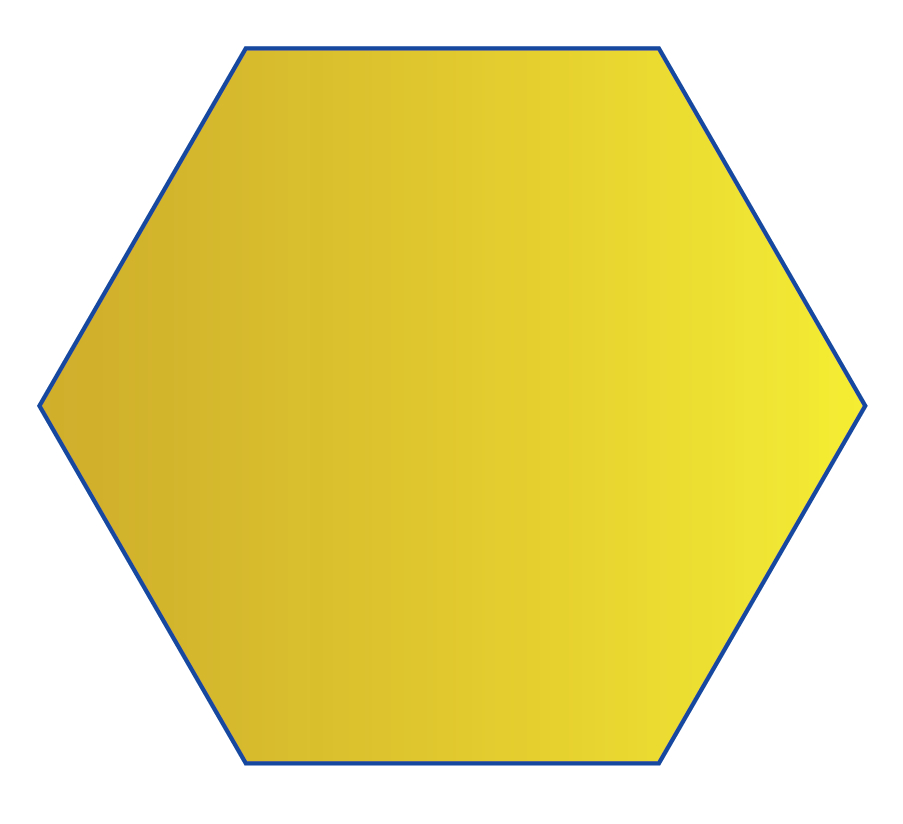Heptagon 7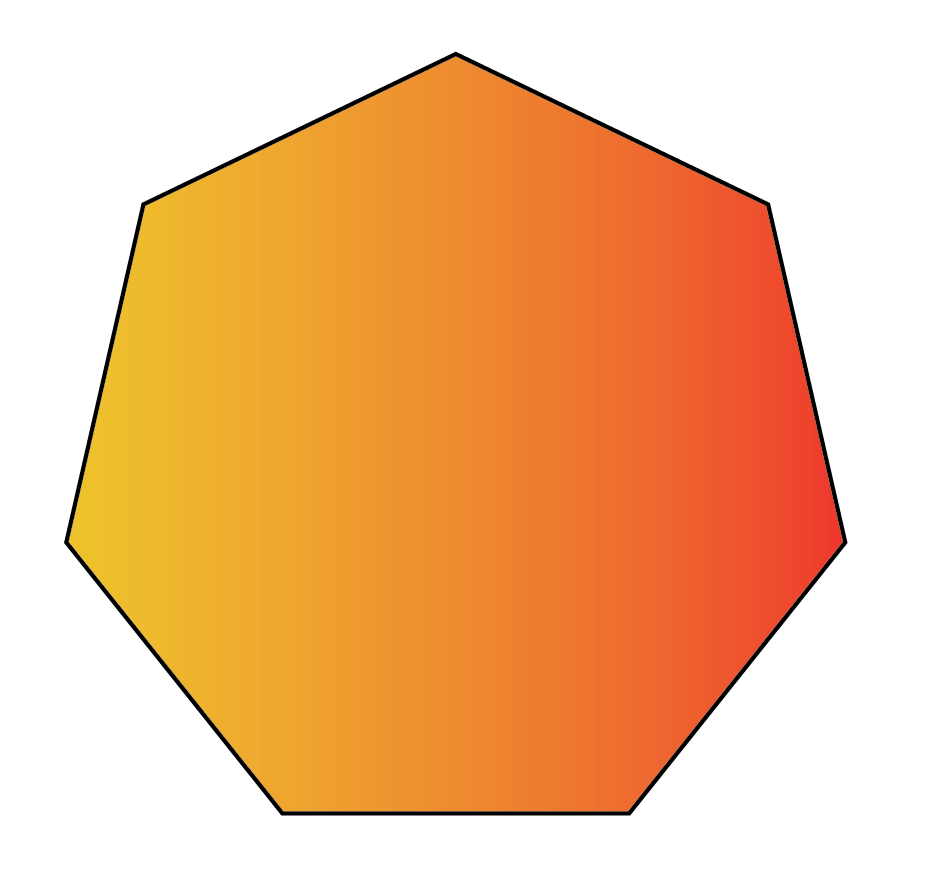Octagon 8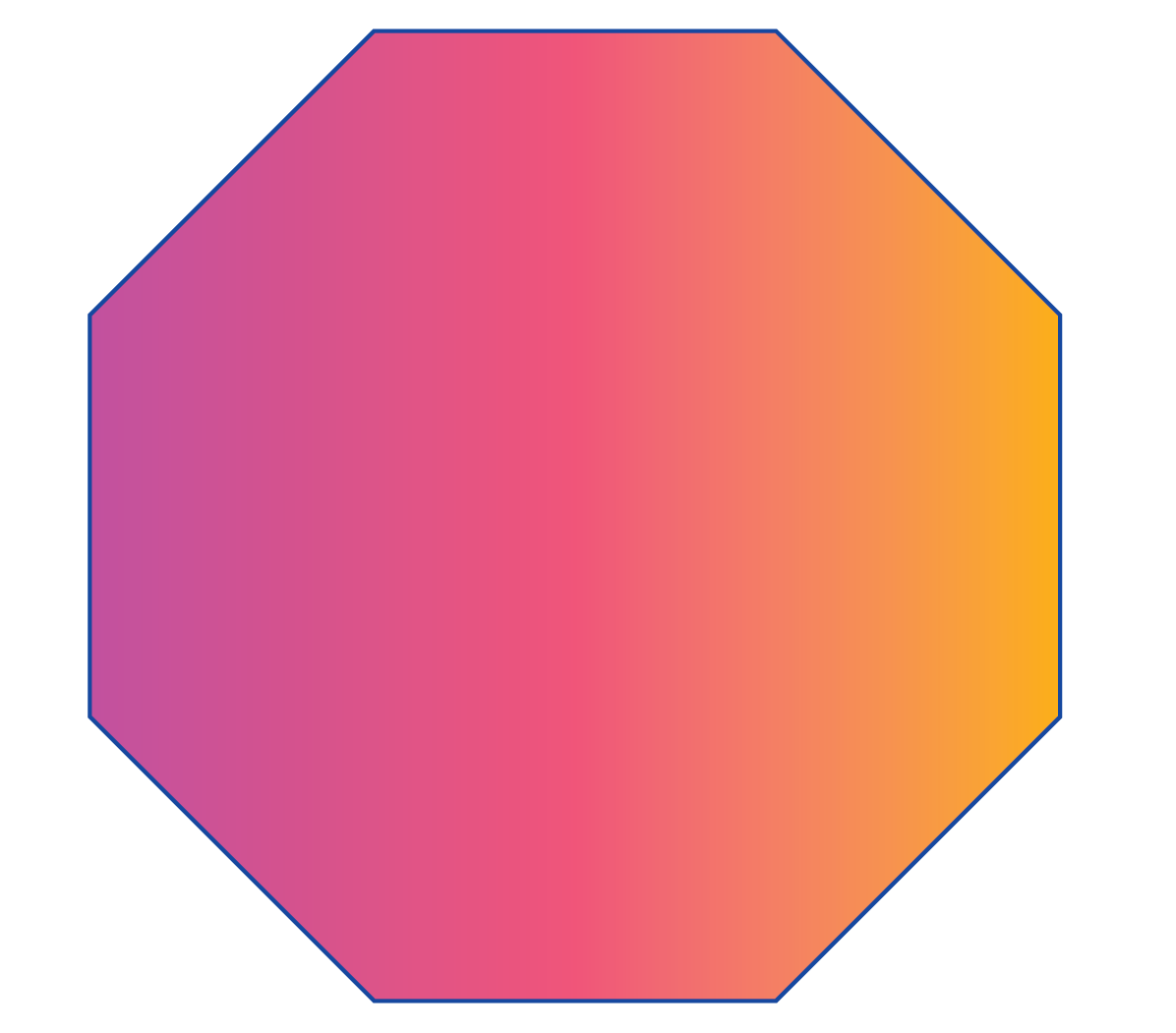Decagon 10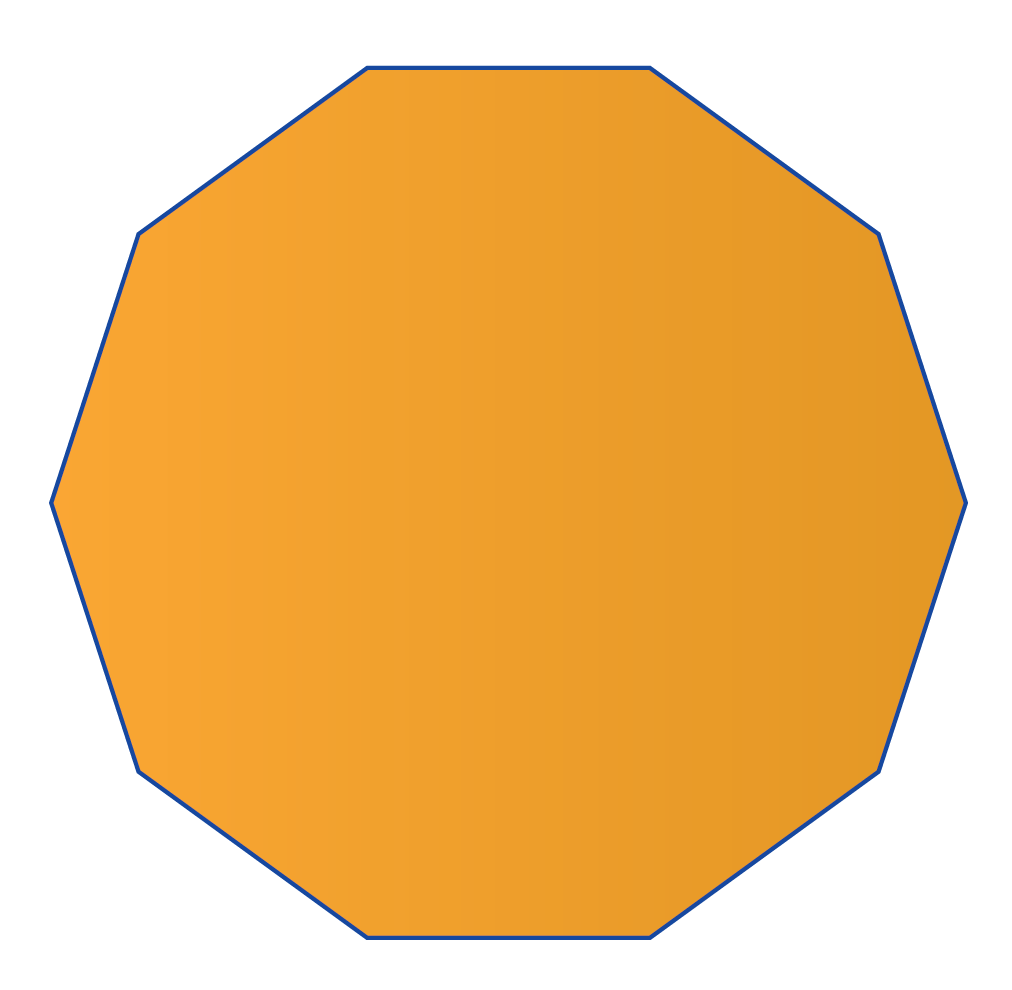Dodecagon 12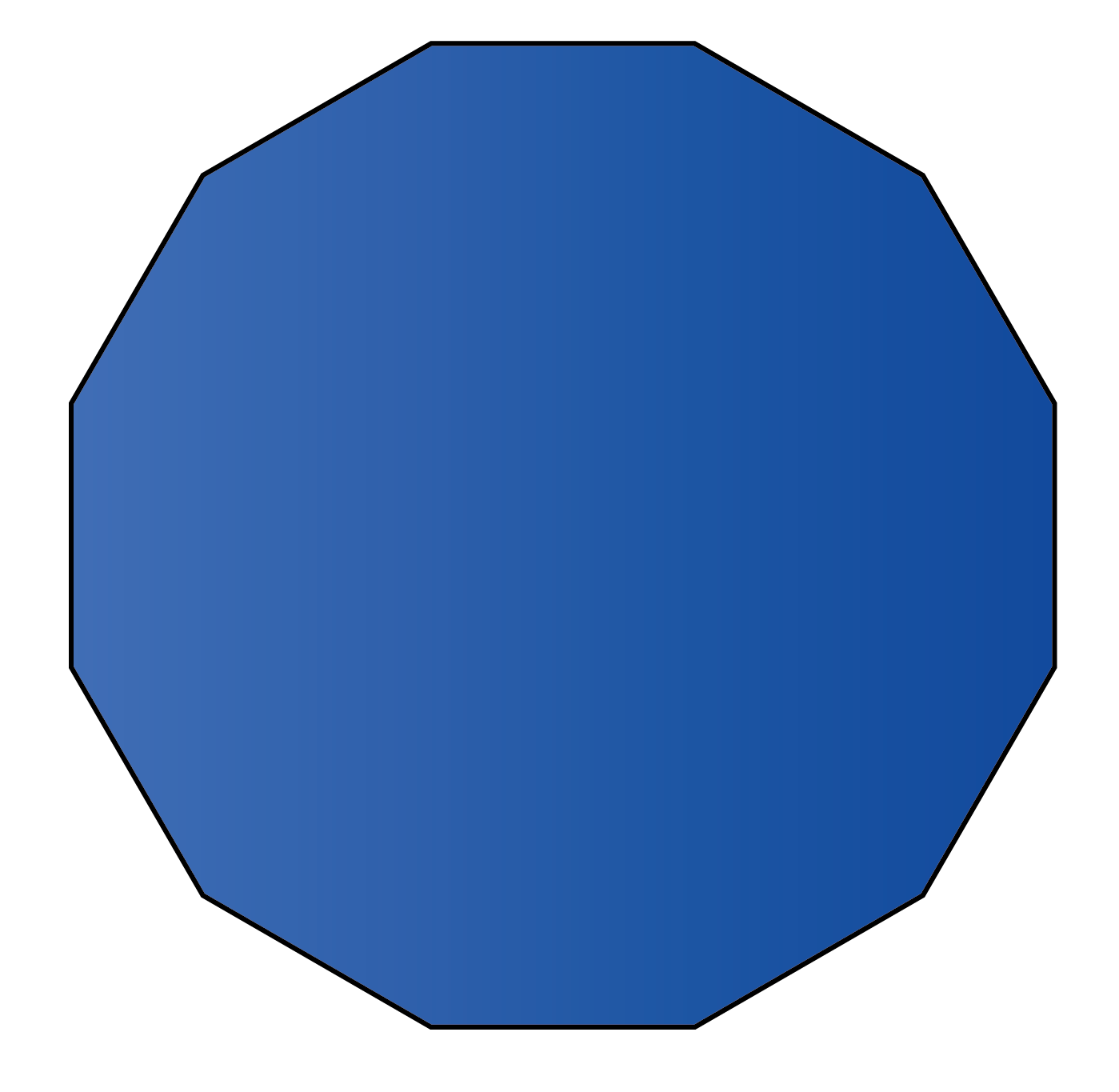Hexadecagon 16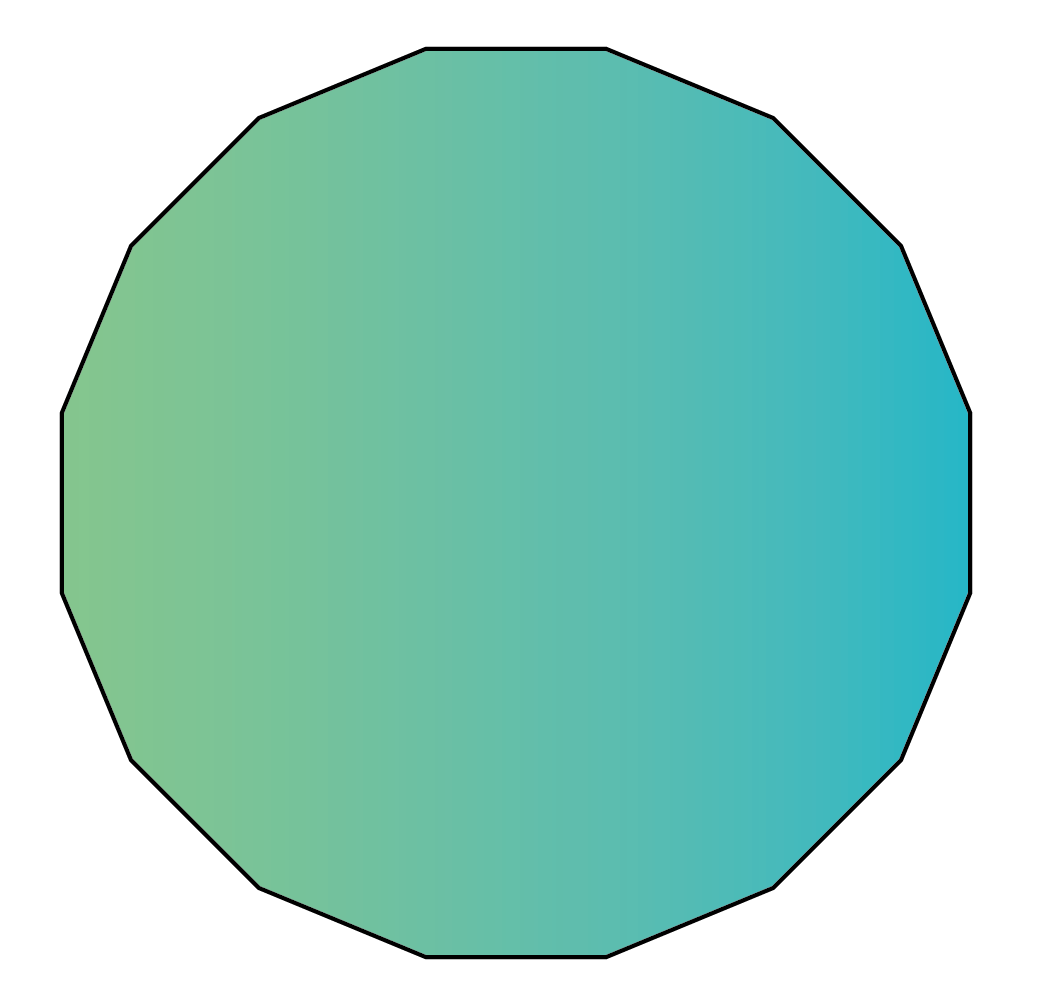Circle 0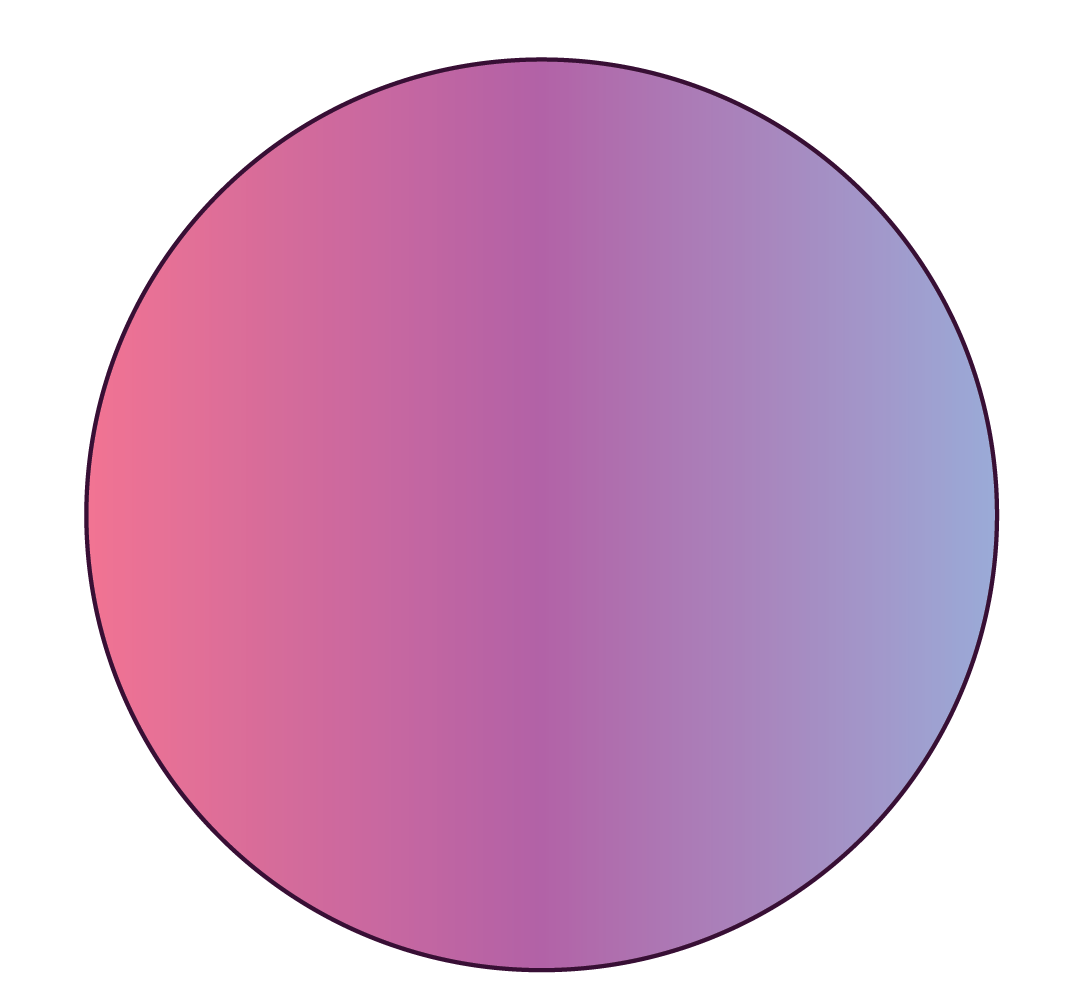Can you draw a few more 2D shapes?

## What are Three-Dimensional Shapes?

Have you seen a dice?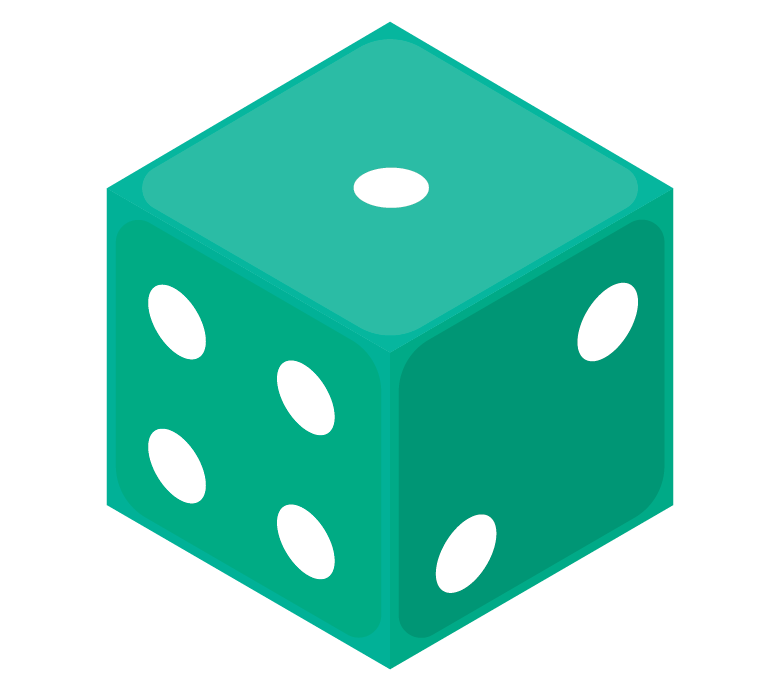This is a three-dimensional shape. It consists of a length, a breadth, and a height.

Three-dimensional shapes have some thickness or depth.

This shape is called a cube. There are several other 3D shapes.

A few are listed here in the table:

Table - 2

 3D Shapes Name Images Cuboid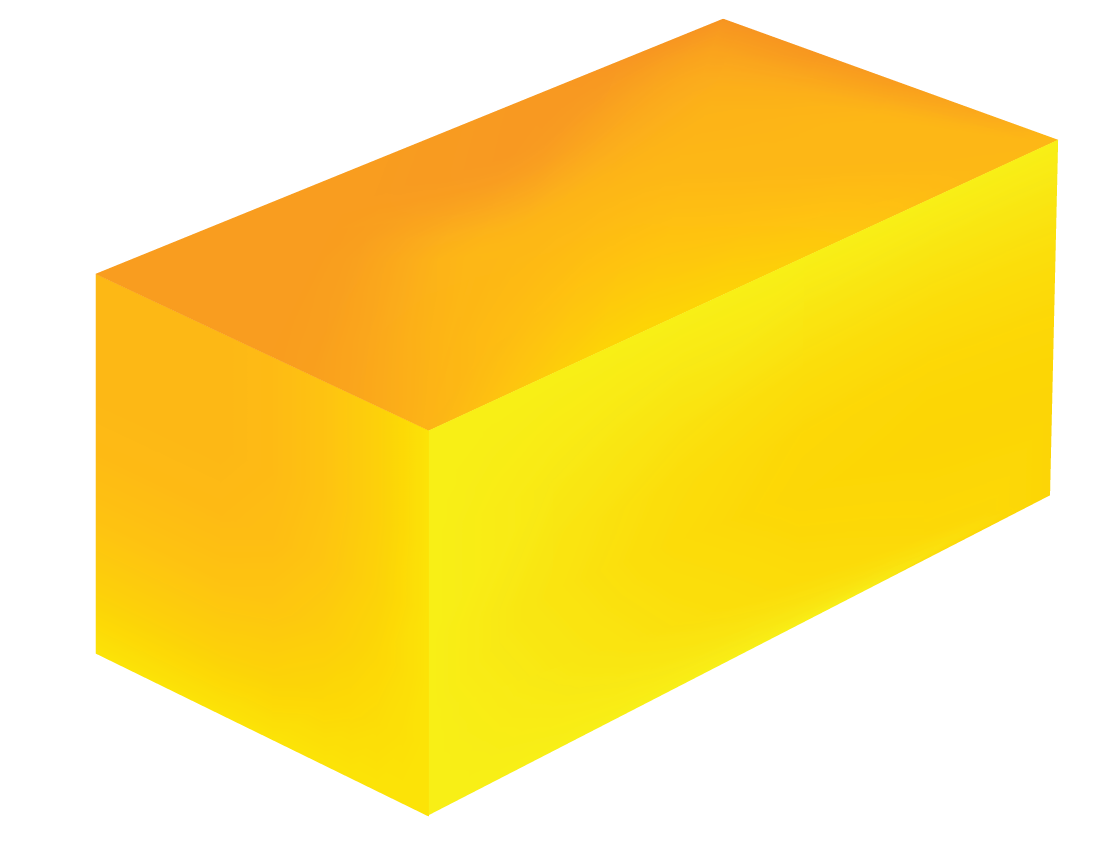Cone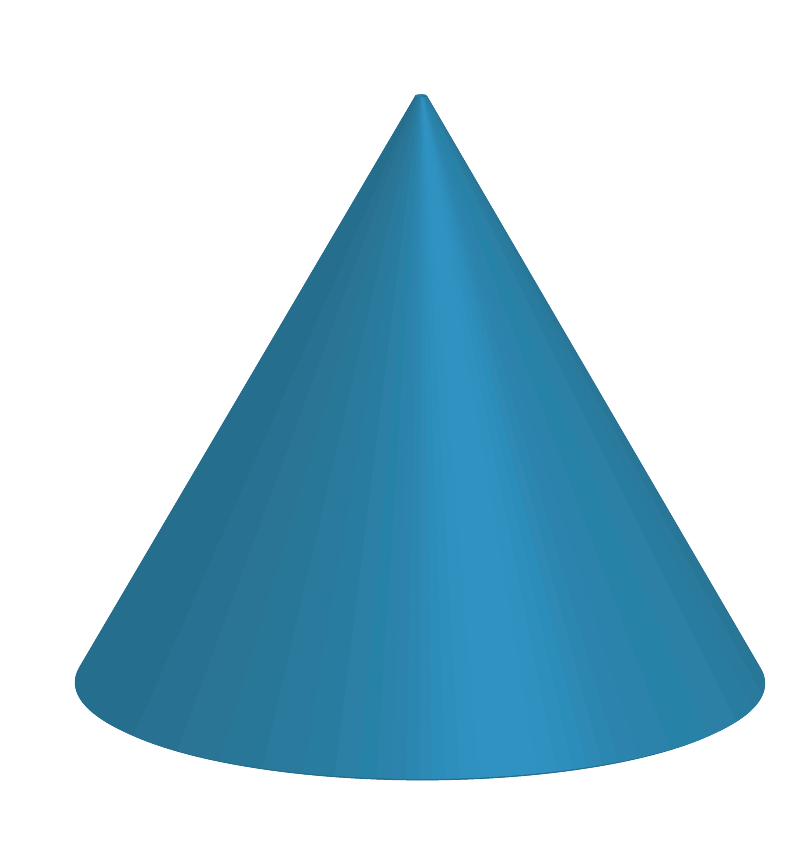Pyramid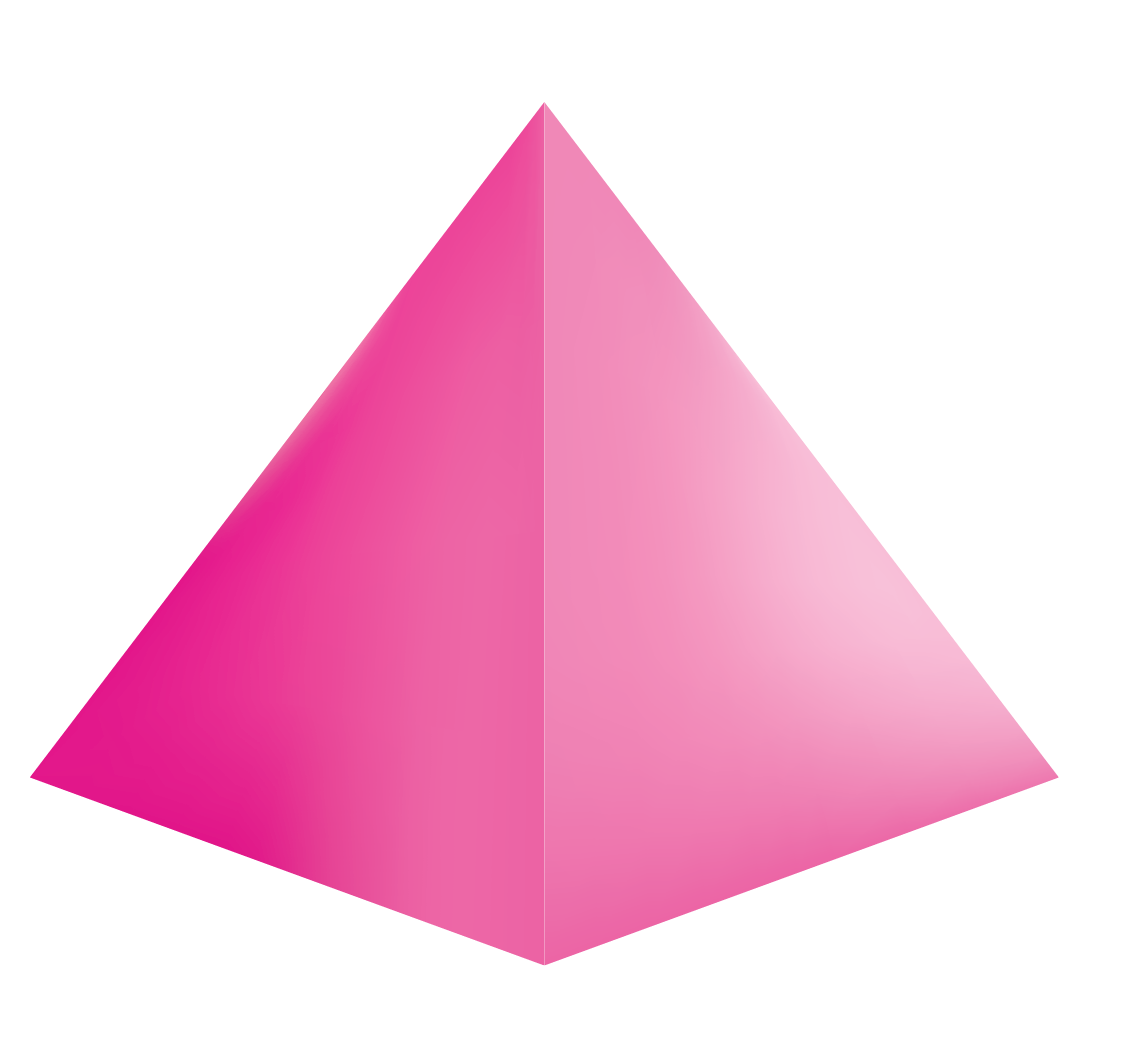Cylinder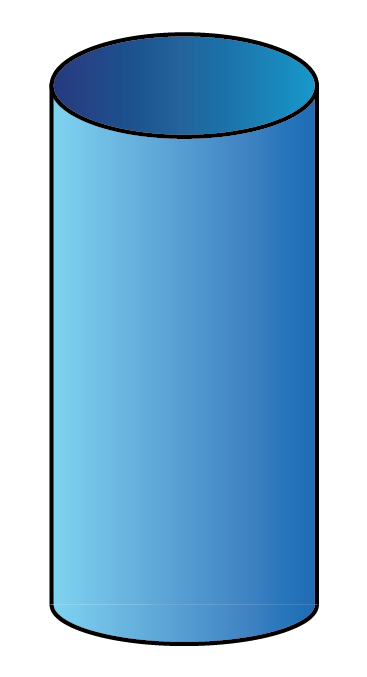Look around you and identify more $$3\text{D}$$ shapes.

More Important Topics
Numbers
Algebra
Geometry
Measurement
Money
Data
Trigonometry
Calculus